# Learning Electronics

Learn to build electronic circuits

# DC branch current analysis

### Question 1:

 Don't just sit there! Build something!!

Learning to mathematically analyze circuits requires much study and practice. Typically, students practice by working through lots of sample problems and checking their answers against those provided by the textbook or the instructor. While this is good, there is a much better way.
You will learn much more by actually building and analyzing real circuits, letting your test equipment provide the änswers" instead of a book or another person. For successful circuit-building exercises, follow these steps:

1.
Carefully measure and record all component values prior to circuit construction.
2.
Draw the schematic diagram for the circuit to be analyzed.
3.
Carefully build this circuit on a breadboard or other convenient medium.
4.
Check the accuracy of the circuit's construction, following each wire to each connection point, and verifying these elements one-by-one on the diagram.
5.
Mathematically analyze the circuit, solving for all values of voltage, current, etc.
6.
Carefully measure those quantities, to verify the accuracy of your analysis.
7.
If there are any substantial errors (greater than a few percent), carefully check your circuit's construction against the diagram, then carefully re-calculate the values and re-measure.

Avoid very high and very low resistor values, to avoid measurement errors caused by meter "loading". I recommend resistors between 1 kW and 100 kW, unless, of course, the purpose of the circuit is to illustrate the effects of meter loading!
One way you can save time and reduce the possibility of error is to begin with a very simple circuit and incrementally add components to increase its complexity after each analysis, rather than building a whole new circuit for each practice problem. Another time-saving technique is to re-use the same components in a variety of different circuit configurations. This way, you won't have to measure any component's value more than once.
Let the electrons themselves give you the answers to your own "practice problems"!

Notes:
It has been my experience that students require much practice with circuit analysis to become proficient. To this end, instructors usually provide their students with lots of practice problems to work through, and provide answers for students to check their work against. While this approach makes students proficient in circuit theory, it fails to fully educate them.
Students don't just need mathematical practice. They also need real, hands-on practice building circuits and using test equipment. So, I suggest the following alternative approach: students should build their own "practice problems" with real components, and try to mathematically predict the various voltage and current values. This way, the mathematical theory "comes alive," and students gain practical proficiency they wouldn't gain merely by solving equations.
Another reason for following this method of practice is to teach students scientific method: the process of testing a hypothesis (in this case, mathematical predictions) by performing a real experiment. Students will also develop real troubleshooting skills as they occasionally make circuit construction errors.
Spend a few moments of time with your class to review some of the "rules" for building circuits before they begin. Discuss these issues with your students in the same Socratic manner you would normally discuss the worksheet questions, rather than simply telling them what they should and should not do. I never cease to be amazed at how poorly students grasp instructions when presented in a typical lecture (instructor monologue) format!
A note to those instructors who may complain about the "wasted" time required to have students build real circuits instead of just mathematically analyzing theoretical circuits:
What is the purpose of students taking your course?
If your students will be working with real circuits, then they should learn on real circuits whenever possible. If your goal is to educate theoretical physicists, then stick with abstract analysis, by all means! But most of us plan for our students to do something in the real world with the education we give them. The "wasted" time spent building real circuits will pay huge dividends when it comes time for them to apply their knowledge to practical problems.
Furthermore, having students build their own practice problems teaches them how to perform primary research, thus empowering them to continue their electrical/electronics education autonomously.
In most sciences, realistic experiments are much more difficult and expensive to set up than electrical circuits. Nuclear physics, biology, geology, and chemistry professors would just love to be able to have their students apply advanced mathematics to real experiments posing no safety hazard and costing less than a textbook. They can't, but you can. Exploit the convenience inherent to your science, and get those students of yours practicing their math on lots of real circuits!

### Question 2:

A transistor is a semiconductor device that acts as a constant-current regulator. For the sake of analysis, transistors are often considered as constant-current sources: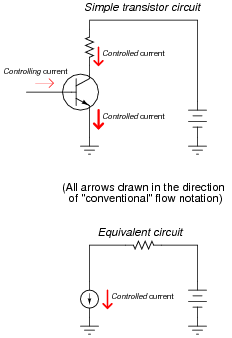Suppose we needed to calculate the amount of current drawn from the 6-volt source in this dual-source transistor circuit: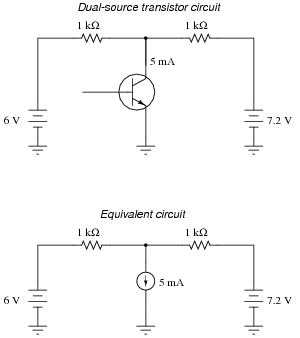We know the combined currents from the two voltage sources must add up to 5 mA, because Kirchhoff's Current Law tells us that currents add algebraically at any node. Based on this knowledge, we may label the current through the 6-volt battery as "I", and the current through the 7.2 volt battery as "5 mA - I":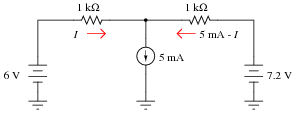Kirchhoff's Voltage Law tells us that the algebraic sum of voltage drops around any "loop" in a circuit must equal zero. Based on all this data, calculate the value of I: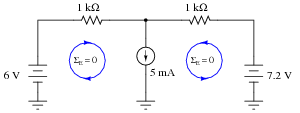Hint: simultaneous equations are not needed to solve this problem!
I = 1.9 mA

Notes:
I wrote this question in such a way that it mimics branch/mesh current analysis, but with enough added information (namely, the current source's value) that there is only one variable to solve for. The idea here is to prepare students for realizing why simultaneous equations are necessary in more complex circuits (when the unknowns cannot all be expressed in terms of a single variable).

### Question 3:

This transistor circuit is powered by two different voltage sources, one that outputs 6 volts, and the other that is variable.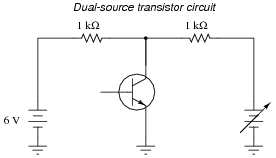Transistors naturally act as current-regulating devices, and are often analyzed as though they were current sources. Suppose that this transistor happened to be regulating current at a value of 3.5 mA: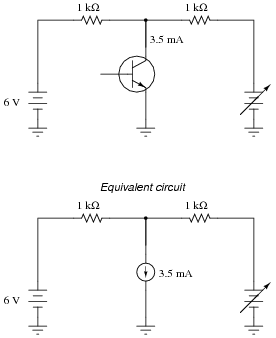How high does the voltage of the variable source have to be adjusted, until no current is drawn from the 6-volt battery?
Hint: simultaneous equations are not needed to solve this problem!
E = 9.5 V

Notes:
The purpose of this question is to get students to apply what they know of basic circuit "laws" (Ohm's Law, KVL, KCL) to the solution of a single voltage value. As usual, the method of solution is far more valuable than the answer.
If some students are completely confused regarding how to solve for this voltage, suggest that they "plug" the given answer into the circuit and determine currents and voltage drops. What do they notice when they do this? What unusual condition(s) stand out with the variable source at 9.5 volts? Are any of these conditions things they could have (or should have) known prior to knowing the variable source's voltage, given the condition of ". . . no current [drawn] from the 6-volt battery"?

### Question 4:

Describe, step-by-step, the steps required to calculate all currents and voltage drops in a DC network using the Branch Current Method.
There are several textbooks and other references delineating the steps required in this analysis method. I leave the task of researching these steps to you!

Notes:
Students may find slight differences between variations of the "Branch Current" method of analysis described in different references. However, these differences are of no consequence.

### Question 5:

While the "Branch Current" method may be used to analyze an unbalanced bridge circuit, it requires a lot of calculation! In this circuit, determine how many variables are needed to solve for all currents:Six variables are required to account for all unique values of current in this circuit (I1 through I6).
Challenge question: draw arrows in this circuit depicting these six currents, and write one KCL equation for each node.

Notes:
Ask your students to explain why it is difficult to solve for the currents in a circuit like this using the "Branch Current" method. How many equations would be necessary to solve for the values of six variables?

### Question 6:

Re-draw the circuit shown here into schematic form, and solve for the voltage drops across the two resistors using the "Branch Current" method: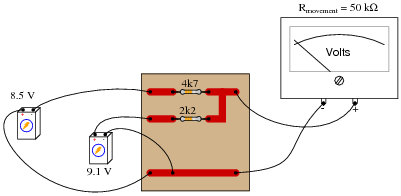E2200 W = 5.713 V
E4700 W = 11.89 V

Notes:
Be sure to spend time with your students comparing their different solution strategies. With there being so many combinations of ways to draw branch currents and write equations, it is highly unlikely that all students' work will be identical. The important lesson here is that different variations still lead to the same (correct) results.

### Question 7:

Calculate the amount of charging current through battery #1 using the Branch Current method of analysis, given the open-circuit voltages and resistances of the components in this circuit. Disregard any wire resistance:Ibat1 = 1.7248 A

Notes:
Ask your students to reveal the equations they used to solve for the current. Their equations will likely differ, but their final answers should be the same!